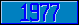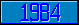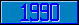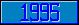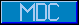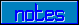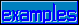## Timer Events

### 7.1.3.3.1 Timer Events:

^\$Event( expr1 V "EVENTDEF" , expr2 V "TIMER" )

^\$Event( "EVENTDEF", "TIMER" ) provides information about the characteristics of the TIMER class of events. Let timerid be the value of a valid evid for a TIMER event. All of the nodes below must be set to establish the timer. If any of these nodes are killed, no timer event occurs.

Timer Event Interval:

^\$Event( expr1 V "EVENTDEF" , expr2 V "TIMER" , timerid , expr3 V "INTERVAL" ) = intexpr

This node identifies (if positive) the running time remaining before the timer event (in seconds). This value counts down continuously at a rate of 1 per second. While the value of this node is greater than 0, the value of the corresponding node ^\$Event( "EVENTDEF", "TIMER", timerid , "ACTIVE" ) (see below) evaluates as a tvexpr to 1.

Timer Event Interval Auto-Reset:

^\$Event( expr1 V "EVENTDEF" , expr2 V "TIMER" , timerid , expr3 V "AUTO" ) = intexpr

This node is the value set into ^\$Event( "EVENTDEF", "TIMER", timerid , "INTERVAL" ) when it is decremented from a positive value to a non-positive value.

Timer Event State:

^\$Event( expr1 V "EVENTDEF" , expr2 V "TIMER" , timerid , expr3 V "ACTIVE" ) = tvexpr

This node identifies the state of the timer. If the node evaluates as a tvexpr to 1, the timer is active (running). If the node evaluates as a tvexpr to 0, the timer is inactive.